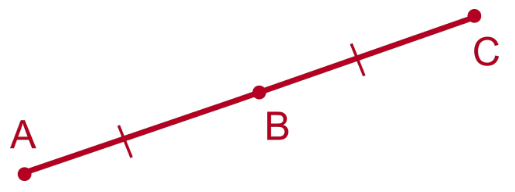# Midpoint Formula

Midpoint formula is used to find the centre point of a straight line. Sometimes you will need to find the number that is half of two particular numbers. For that, you find the average of the two numbers. In that similar fashion, we use the midpoint formula in coordinate geometry to find the halfway number (i.e. point) of two coordinates.## Formula to Find the Midpoint

To find the midpoint of the straight line in a graph, we use this midpoint formula that will enable us to find the coordinates of the midpoint of the given line. Suppose the endpoints of the line are (x1, y2) and (x2, y2) then the midpoint is calculated using the formula given below.

The Midpoint Formula is given as,

 (x, y) = [(x1 + x2)/2, (y1 + y2)/2]

Where, x1, x2 are the coordinates of the x-axis. y1, y2 are the coordinates of the y-axis.

### Solved Examples

Question 1: Find the midpoint of a line whose endpoints are (4, 5) and (6, 7).

Solution:

Given, (x1, y1) = (4, 5)

(x2, y2) = (6, 7)

According to the formula we can find the midpoint (x, y):

(x, y) = [(x1 + x2)/2, (y1 + y2)/2]

(x, y) = [(4 + 6)/2, (5 + 7)/2]

= (5, 6)

Question 2: If (1, 0) is the midpoint of the line joining the points A(-6, -5) and B, then find the coordinates of B.

Solution:
Given,
(1, 0) is the midpoint of A and B.
A = (-6, -5) = (x1, y1)
Let the coordinates of B are (a, b).
(a, b) = (x2, y2)
Using the midpoint formula,
(x, y) = [(x1 + x2)/2, (y1 + y2)/2] (1, 0) = [(a – 6)/2, (b – 5)/2]

Now equating the x and y coordinates,
(a – 6)/2 = 1, (b – 5)/2 = 0
a – 6 = 2, b – 5 = 0
a = 8, b = 5
Therefore, the coordinates of B = (8, 5).

Question 3: Find the coordinates of the centre of the circle whose endpoints of a diameter are (0, 2), (3, 4).
Solution:
Given coordinates of endpoints of a diameter are:
(0, 2) = (x1, y1)
(3, 4) = (x2, y2)
Centre of the circle is the midpoint of diameter.
Coordinates of the centre of a circle = [(x1 + x2)/2, (y1 + y2)/2]

= [(0 + 3)/2, (2 + 4)/2]

= (3/2, 6/2)

= (1.5, 3)